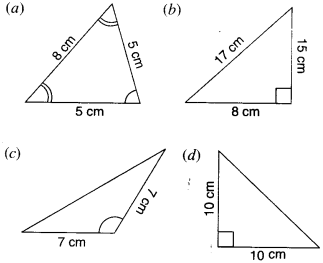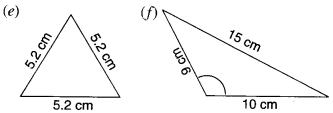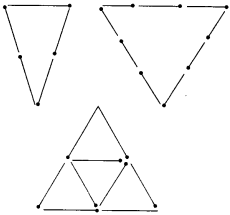NCERT Solutions for Class 6 Maths Chapter 5 Understanding Elementary Shapes Ex 5.6 are part of NCERT Solutions for Class 6 Maths. Here we have given NCERT Solutions for Class 6 Maths Chapter 5 Understanding Elementary Shapes Ex 5.6.

 Board CBSE Textbook NCERT Class Class 6 Subject Maths Chapter Chapter 5 Chapter Name Understanding Elementary Shapes Exercise Ex 5.6 Number of Questions Solved 4 Category NCERT Solutions

## NCERT Solutions for Class 6 Maths Chapter 5 Understanding Elementary Shapes Ex 5.6

Question 1.
Name the types of following triangles:
(a) Triangle with lengths of sides 7 cm, 8 cm and 9 cm.
(b) ΔABC with AB = 8.7cm, AC =7cm andBC = 6 cm.
(c) ΔPQR such that PQ = QR = PR = 5 cm.
(d) ΔDEF with m ∠D = 90°.
(e) ΔXYZ with m ∠Y = 90° and XY = YZ.
(f) ΔLMN with m ∠L = 30°, m ∠M =70° and m ∠N = 80°.
Solution :
(a) Scalene triangle
(b) Scalene triangle
(c) Equilateral triangle
(d) Right angled triangle
(e) Isosceles right triangle
(f) Acute angled triangle.

Question 2.
Match the following :

 Measures of triangle Type triangle (i) 3 sides of equal length (a) Scalene (ii) 2 sides of equal length (b) Isosceles right angled (iii) All sides are of different length (c) Obtuse angled (iv) 3 acute angles (d) Right angled (v) 1 right angle (e) Equilateral (vi) 1 obtuse angle (f) Acute-angled (vii) 1 right angle with two sides of equal length (g) Isosceles

Solution :

 Measures of triangle Type triangle (i) 3 sides of equal length (e) Equilateral (ii) 2 sides of equal length (g) Isosceles (iii) All sides are of different length (a) Scalene (iv) 3 acute angles (f) Acute-angled (v) 1 right angle (d) Right angled (vi) 1 obtuse angle (c) Obtuse angled (vii) 1 right angle with two sides of equal length (b) Isosceles right angled

Question 3.
Name each of the following triangles in two different ways : (you may judge the nature of the angle by observation).Solution :
(a) Acute-angled and isosceles.
(b) Right-angled and scalene.
(c) Obtuse-angled and isosceles.
(d) Right-angled and isosceles.
(e) Equilateral and acute-angled.
(f) Obtuse-angled and scalene.

Question 4.
Try to construct triangles using matchsticks. Some are shown here. Can you make a triangle with(a) 3 matchsticks?
(b) 4 matchsticks?
(c) 5 matchsticks?
(d) 6 matchsticks?
(Remember you have to use all the available matchsticks in each case). Name the type of triangle in each case. If you cannot make a triangle, think of reasons for it.
Solution :
(a) Yes, an equilateral triangle.
(b) No, since the sum of the lengths of any two sides of a triangle is always greater than the length of the third side.
(c) Yes, an isosceles triangle.
(d) Yes, an equilateral triangle.

We hope the NCERT Solutions for Class 6 Maths Chapter 5 Understanding Elementary Shapes Ex 5.6 help you. If you have any query regarding NCERT Solutions for Class 6 Maths Chapter 5 Understanding Elementary Shapes Ex 5.6, drop a comment below and we will get back to you at the earliest.